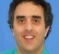## Correlation of complex sinusoidStarted by 5 years ago6 replieslatest reply 5 years ago255 views

Hi,

Feel a bit sheepish about not being able to figure this out on my own.

I am playing with correlating known sequences/preambles in a wireless signal. If my template signal is a sinusoid, what would be autocorrelation output?

For real signals, (I think) I know that the auto-correlation of a sinusoid is a  (sinusoidal) function of the time/phase difference of the sinusoid, which is intuitive i.e. when the received signal and the template are in phase, one would see a correlation peak, when they are completely out of phase, one would see the minimum correlation output, and so on.

For complex signals, does this also hold? I would think so, but my signal processing software (X-midas) is giving me a flat constant output when I slide the template signal over the received signal. I would have expected the output to be a sinusoid with peaks when the template is exactly aligned with the received signal, and not a flat/constant value.

Wanted to confirm I am not missing something basic before trying to figure out if the software routine is doing somethign wrong.

Thanks

[ - ]The absolute value of your correlation will always be 1, but the phase will change. Maybe your software just shows you the absolute value?

[ - ]Yes - it shows the absolute value. Thanks.

[ - ]I think the best way think about complex correlation is from a geometric viewpoint. If you represent each complex sinusoid as rotating phasor on the complex plane the correlation is represented as the orthogonal projection of one vector onto the other. So for phasors that are in phase the correlation would be +1 (positive correlation), for phasors that are 90 degrees out of phase the correlation would be 0 (uncorrelated) and for phasors that are 180 degrees out of phase the correlation would be -1 (negative correlation).

[ - ]Hi Jw,

I was thinking along those lines too, but that doesn't line up with the constant correlation I get in software (and as said by dudelsound). I suppose for complex sinusoids no matter what the phase difference between the two waveforms being correlated, the correlation (magnitude) is always 1/constant.

[ - ]